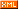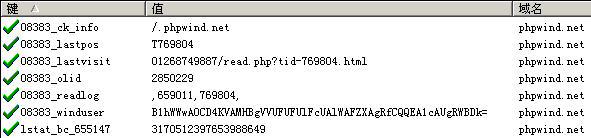# 琢思磋文轩

 博客园 :: 首页 :: 博问 :: 闪存 :: 新随笔 :: 联系 :: 订阅:: 管理 ::JSP网站与phpwind论坛同步登入的解决思路Cookie("winduser",StrCode($winduid."\t".$windpwd."\t".$safecv),$cktime);

function Cookie($ck_Var,$ck_Value,$ck_Time='F',$p=true){ }

!isset($pre) &&$pre = substr(md5($GLOBALS['db_sitehash']),0,5); return$pre;

}

$logininfo = checkpass($pwuser, $md5_pwpwd,$safecv, $lgt); list($winduid, $groupid,$windpwd, $showmsginfo) =$logininfo;

function checkpass($username,$password, $safecv,$lgt=0) { }

$windpwd = PwdCode($password);

return md5($GLOBALS['pwServer']['HTTP_USER_AGENT'].$pwd.$GLOBALS['db_hash']); } 这样就明白了，$windpwd其实就是Request请求中的User-Agent头信息的字符串、用md5加密过的用户密码、pw_config表中数据db_hash所对应值，三者依次拼接而成的字符串进行一次md5加密之后所产生的字符串，真是够复杂的，看来phpwind为了安全也是煞费苦心啊！

$question =$question=='-1' ? $customquest :$question;

return $question ? substr(md5(md5($question).md5($answer)),8,10) : ''; }$safecv的产生过程也够复杂的，相信大家通过上面的讲解也能明白这个产生过程了，那么笔者也就不多费口舌，太累人了！

list($winduid,$windpwd,$safecv)=explode("\t",addslashes(StrCode(GetCookie('winduser'),'DECODE'))); 其重点在于StrCode(GetCookie('winduser'),'DECODE')，作用是将从Cookie中获取的值采用base64方式进行解码。解码之后的字符串用"\t"分割为三段，第一段是uid，第二段是由上文所提到的PwdCode函数生成的字符串，第三段是由上文所提到的questcode函数生成的字段串，分别存入$winduid$windpwd$safecv中。之后就可以通过该uid来获取用户信息，并验证相关的密码与安全问题是否一致了。

三、实现与phpwind论坛同步登入和同步退出的思路